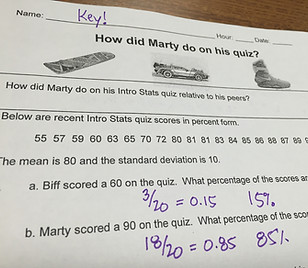top of page

## Day 12 - Lesson 1.9

##### Learning Targets
• Find and interpret a percentile in a distribution of quantitative data.

• Estimate percentiles and individual values using a cumulative relative frequency graph.

• Find and interpret a standardized score (z-score) in a distribution of quantitative data.

##### Activity:

Sometimes it’s better to give students a data set rather than to collect their own.  With this activity, we needed to be sure that the data set would give us a standard deviation that was easy for students to compute with.  So when we are not having students collect relevant data, we still try to make it memorable with a theme.  This time we went with Back to the Future!

The goal of this activity was to give students an understanding of what a percentile and z-score tell us.  We are very focused on the students being able to accurately interpret, more so than on their ability to calculate.  This activity went really well.  Students were able to quickly figure out how many standard deviations away from the mean Marty and Biff’s scores were.  When the score was not a full standard deviation away, students had little issue finding the number of standard deviations as a decimal.  After they completed the page, we had them write in the margins that the percents they found in the first question were really percentiles.  We also wrote the z-scores in the margin for each of the questions they had answered.##### Application 1.9

Students had a bit of a hard time with the cumulative relative frequency graphs.  Who can blame them with a name like that! To help with this we have been referring to it as a percentile graph.  We label the vertical axis percentile every time we use one of these graphs and that was helpful.  The groups seemed ok with finding the median and quartiles in class but when they took their quiz and test they really struggled.  Instead of finding the median using the 50th percentile on the vertical axis, they instead found the middle of the horizontal axis and said that was the median.

Bonus: If you have time, try the online only Lesson 1.9A on Transforming Data.

bottom of page